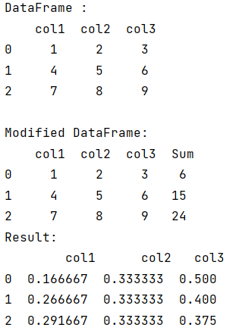# Python - Pandas sum across columns and divide each cell from that value

Given a Pandas DataFrame, we have to find the sum across columns and divide each cell from that value.
Submitted by Pranit Sharma, on August 04, 2022

Pandas is a special tool that allows us to perform complex manipulations of data effectively and efficiently. Inside pandas, we mostly deal with a dataset in the form of DataFrame. DataFrames are 2-dimensional data structures in pandas. DataFrames consist of rows, columns, and data.

Sometimes, we need to sum across a specific range of columns by each row and divide each cell by the sum of that row.

Pandas library consists of almost every kind of logical and mathematical function which are required for data analysis. With the help of the pandas library, we can easily draw useful insights from the data set that we are working on.

To sum across columns and divide each cell from that value, we will first create a Dataframe then we will apply the sum() method to find the sum row-wise. Then we will use the div() method for dividing each cell from the sum value.

Let us understand with the help of an example,

## Python code to find the sum across columns and divide each cell from that value

```# Importing pandas package
import pandas as pd

# Creating a dictionary
d = {
'col1':[1,4,7],
'col2':[2,5,8],
'col3':[3,6,9]
}

# Creating a dataframe
df = pd.DataFrame(d)

# Display Dataframe
print("DataFrame :\n",df,"\n")

# Applying sum function
df["Sum"] = df.sum(axis=1)

# Display modified DataFrame
print("Modified DataFrame:\n",df)

# Using div function
result = df.loc[:,"col1":"col3"].div(df["Sum"], axis=0)

# Display result
print("Result:\n",result)
```

Output:Languages: » C » C++ » C++ STL » Java » Data Structure » C#.Net » Android » Kotlin » SQL
Web Technologies: » PHP » Python » JavaScript » CSS » Ajax » Node.js » Web programming/HTML
Solved programs: » C » C++ » DS » Java » C#
Aptitude que. & ans.: » C » C++ » Java » DBMS
Interview que. & ans.: » C » Embedded C » Java » SEO » HR
CS Subjects: » CS Basics » O.S. » Networks » DBMS » Embedded Systems » Cloud Computing
» Machine learning » CS Organizations » Linux » DOS
More: » Articles » Puzzles » News/Updates